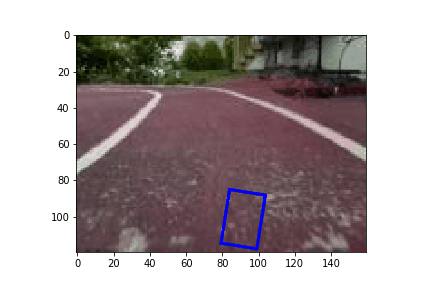# 《制作无人驾驶车辆》第 4 部分：通过 Apache MXNet，为您的自动驾驶车辆应用行为克隆技术

1) 在 AWS 上制造一辆无人驾驶车辆，并参加 re:Invent 机器人车拉力赛
2) 《制作无人驾驶车辆》第 2 部分：驾驶您的车辆
3) 《制作无人驾驶车辆》第 3 部分：连接您的无人驾驶车辆
4) 《制作无人驾驶车辆》第 4 部分：通过 Apache MXNet，为您的自动驾驶车辆应用行为克隆技术

## P2 上的 Donkey 训练数据设置

1. 将数据从 Pi 复制到 Amazon EC2 实例：
`\$ rsync -rva --progress -e "ssh -i /path/to/key/DonkeyKP-us-east-1.pem" /home/pi/d2// ec2-user@ec2-your-ip.compute-1.amazonaws.com:~/d2/data/`
2. 启动训练过程：
`\$ python ~/d2/manage.py train --model /path/to/myfirstpilot`
3. 将训练过的模型复制回 Pi：
`\$: rsync -rva --progress -e "ssh -i /path/to/key/DonkeyKP-us-east-1.pem" ec2-user@ec2-your-ip.compute-1.amazonaws.com:~/d2/models/ /home/pi/d2/models/`

## 模型幕后

### 制作 CNN 模型

```import mxnet as mx
import numpy as np

data = mx.symbol.Variable(name="data")

body = mx.sym.Convolution(data=data, num_filter=24,  kernel=(5, 5), stride=(2,2))
body = mx.sym.Activation(data=body, act_type='relu', name='relu1')
body = mx.symbol.Pooling(data=body, kernel=(2, 2), stride=(2,2), pool_type='max')

body = mx.sym.Convolution(data=body, num_filter=32,  kernel=(5, 5), stride=(2,2))
body = mx.sym.Activation(data=body, act_type='relu')
body = mx.symbol.Pooling(data=body, kernel=(2, 2), stride=(2,2), pool_type='max')

flatten = mx.symbol.Flatten(data=body)

body = mx.symbol.FullyConnected(data=flatten, name='fc0', num_hidden=32)
body = mx.sym.Activation(data=body, act_type='relu', name='relu6')
body = mx.sym.Dropout(data=body, p=0.1)

body = mx.symbol.FullyConnected(data=body, name='fc1', num_hidden=16)
body = mx.sym.Activation(data=body, act_type='relu', name='relu7')

out = mx.symbol.FullyConnected(data=body, name='fc2', num_hidden=1)
out = mx.symbol.LinearRegressionOutput(data=out, name="softmax")
```

### 训练模型

```# Get Iterators

def get_iterators(batch_size, data_shape=(3, 120, 160)):
train = mx.io.ImageRecordIter(
path_imgrec         = 'train.rec',
data_name           = 'data',
label_name          = 'softmax_label',
batch_size          = batch_size,
data_shape          = data_shape,
shuffle             = True,
rand_crop           = True,
rand_mirror         = True)
val = mx.io.ImageRecordIter(
path_imgrec         = 'valid.rec',
data_name           = 'data',
label_name          = 'softmax_label',
batch_size          = batch_size,
data_shape          = data_shape,
rand_crop           = False,
rand_mirror         = False)
return (train, val)

batch_size = 16
train_iter, val_iter = get_iterators(batch_size)

#Training

batch_size = 8
num_gpus = 1
num_epoch = 10
mod = mx.mod.Module(out, context=[mx.gpu(i) for i in range(num_gpus)])
mod.fit(train_data=train_iter,
eval_data=val_iter,
eval_metric='mae',
optimizer_params={'learning_rate': 0.0001},
num_epoch=num_epoch,
batch_end_callback = mx.callback.Speedometer(batch_size, 100),
)```

### 评估和模拟器

```import os
import time
%matplotlib inline
from IPython import display
import matplotlib.patches as patches

PATH = 'trainingdata/'
all_files = sorted(os.listdir(PATH))
sym, arg_params, aux_params = mx.model.load_checkpoint('my-car', num_epoch)
mod = mx.mod.Module(symbol=sym)
fig, ax = plt.subplots(1)
for fname in all_files:
org_img = Image.open(PATH + fname)
img = np.array(org_img)
img = np.swapaxes(img, 0, 2)
img = np.swapaxes(img, 1, 2)
img = img[np.newaxis, :]
mod.forward(Batch(data=[mx.nd.array(img)]))
exp = mod.get_outputs().asnumpy()
angle = 180*exp
left = 80 * exp + 80
rect = patches.Rectangle((left, 85),20,30, angle=angle,
linewidth=2,edgecolor='r',facecolor='none')